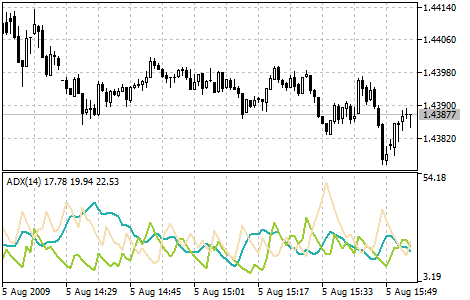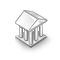Interesting script?
So post a link to it -
let others appraise it

You liked the script? Try it in the MetaTrader 5 terminalViews:
25305
Rating:
Published:
2010.01.06 17:21
Updated:
2016.11.22 07:32

Average Directional Movement Index technical indicator (ADX) helps to determine the market trend. It was developed and described in detail by Welles Wilder in his book "New concepts in technical trading systems".

The simplest trading method based on the system of directional movement implies comparison of two direction indicators: the 14-period +DI one and the 14-period -DI. To do this, one either puts the charts of indicators one on top of the other, or +DI is subtracted from -DI. W. Wilder recommends buying whenever the +DI crosses above the -DI, and selling when -DI crosses above the +DI.

In addition to these simple commercial rules, Wells Wilder offered the extreme point rule. It is used to eliminate false signals and decrease the number of deals. According to the principle of extreme points, the "extreme point" occurs when +DI and -DI cross each other. If +DI raises higher than -DI, this point will be the maximum price of the day when they cross. If +DI is lower than -DI, this point will be the minimum price of the day they cross.

The extreme point is used then as the market entry level. Thus, after the signal to buy (+DI is above -DI) one must wait till the price exceeds the extreme point, and only then buy. However, if the price fails to exceed the level of the extreme point, one should retain the short position.Calculation:

ADX = SUM ((+DI - (-DI)) / (+DI + (-DI)), N) / N

where:

• N - the number of periods used in the calculation;
• SUM (..., N) - sum for N periods;
• +DI - value of the indicator of the positive price movement (positive directional index);
• -DI - value of the indicator of the negative price movement (negative directional index).

Translated from Russian by MetaQuotes Software Corp.
Original code: https://www.mql5.com/ru/code/7Accelerator Oscillator (AC)

The Acceleration/Deceleration Indicator (AC) measures acceleration and deceleration of the current driving force.MACD Signals

Indicator edition for new platform.Class CalcFrac - Number of bars before and after the current High / Low (calculated fractal)

Calculates fractals and lets you specify the number of bars separately before and after the current High / Low (fractal).Average Directional Movement Index Wilder

The Average Directional Movement Index by Wilder (ADX Wilder) helps to determine the presence of the price trend.